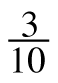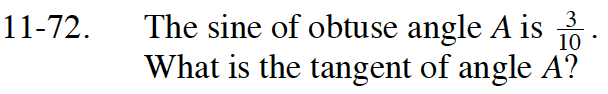### Home > INT3 > Chapter Ch11 > Lesson 11.2.2 > Problem11-72

11-72.

The sine of obtuse angle A is. What is the tangent of angle A? Homework Help ✎First use sin2A + cos2A = 1 to find cosA.
Note that A is in the second quadrant.

$\text{tan}A=\frac{-3}{\sqrt{91}}$